Technical Article

# Research of Properties of Manganese Zinc Ferrites with Fepoor Composition

August 31, 2018 by YangZhong Du

## With the continuous development of electronic technology, especially the rapid development of digital technology, the world put forward high requirements

With the continuous development of electronic technology, especially the rapid development of digital technology, the world put forward high requirements on resistance to electromagnetic interference technology of electronic device (EMI), which requiring materials used for these devices should have excellent EMI property. Commonly used EMI ferrite materials have MnZn materials and NiZn materials. Currently, MnZn ferrite material on the market is Fe-rich composition formula, that is to say the proportion of Fe2O3 in the three main raw materials is more than 50mol%. According to the composition diagram of MnZn ferrite permeability (Figure 1), Fe3+-short composition can also obtain high permeability. In this paper, we select several Fe-poor composition formulas to conduct a relevant experiment and study their related properties.Figure 1. Composition diagram

## Experimental Method

According to the composition diagram of MnZn ferrite and the influence of zinc content on the Curie temperature, the authors of this paper chose four compositions as shown in Table 1:

Table 1. Formulation Composition## Results of the Fe-poor Composition Formula Experiment

### The Influence of ZnO Content on the Initial Permeability of Materials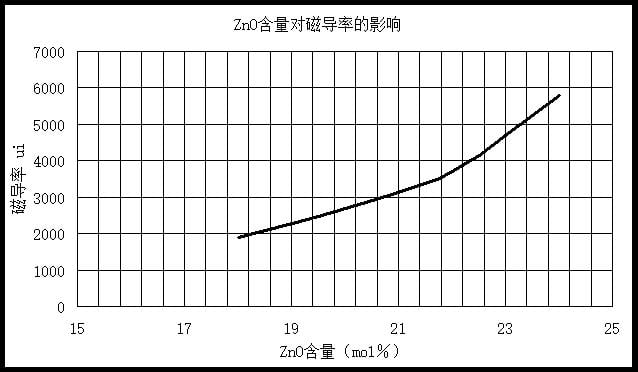Figure 2. The influence of ZnO content on permeability

According to the formula chosen in this paper, fix Fe2O3 content 49.5 mol %, change ZnO content. As shown in Table 1, 4 different formula ring cores after sintered in certain conditions, permeability results as shown in Figure 2.

As can be seen from Figure 2, with the increase of ZnO content, permeability shows an uptrend. When the ratio is Fe2O3 49.5mol%, ZnO 24mol%, the permeability of the ring core can reach around 6000. According to magnetocrystalline anisotropy constant K1 curve (Figure 3), K1 of Fe-poor composition formula selected by this paper is less than 0. With the increase of ZnO content, K1 gets closer to 0, so you get higher permeability.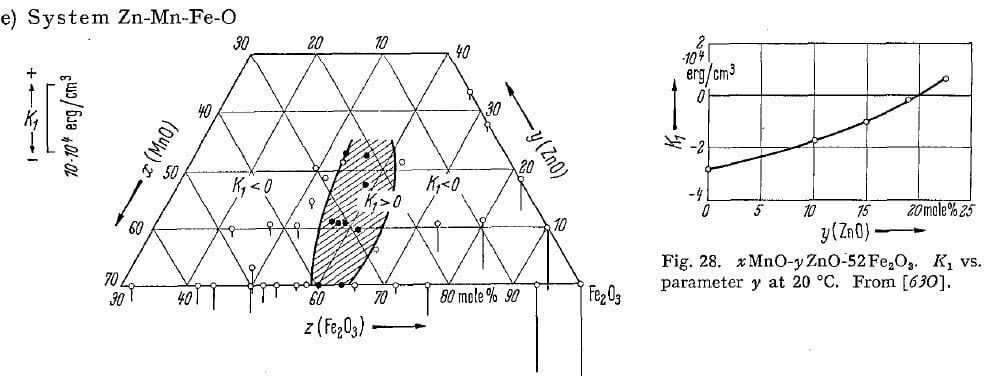Figure 3. K1 vs MnZn composition diagram

### Influence of ZnO on Curie Temperature

According to related literature, theoretical calculation of the Curie temperature of Fe-poor composition formula can be calculated with the following formula:

Tc = 6.475*{ X - (2/3)*Z } - 104 [ °C ]

X represents Fe2O3 mol%, Z represents ZnO mol%, comparisons of the theoretical Curie temperature according to the calculation formula, and the actual testing results of the Curie temperature are shown in Table 2.

Table 2. Comparisons of the Theoretical Curie Temperatures and the Actual Testing Curie Temperatures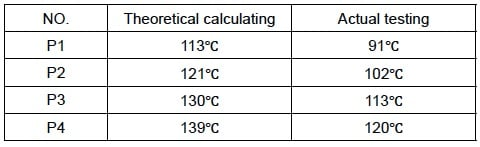From the above comparison, it can be seen that the actual Curie temperature has some deviation from the theoretical calculation of the Curie temperature, which is related to the sintering process and the certain addition of additives. Theoretical calculation formula of Curie temperature under certain conditions needs modification.

According to the formula of Curie temperature, with the increase of ZnO content, Curie temperature of material will decrease. When ZnO content reaches 24mol% and Curie temperature is around 90°C, for actual use it makes no sense. Therefore, when choosing a formula, it is necessary to consider the actual application and choose the proper proportion of the main raw materials.

### A Comparison of Fe-rich Grades with the Same Permeability

We performed comparison tests of the u’/u’’~f, u~T, impedance, and resistivity characteristics of the Fe-rich composition formula and the Fe-poor composition formula samples with a permeability of around 4000.

From Figures 4 and 5 we can see that the Fe-poor composition formula samples have better properties at high frequency. What’s more, impedance is higher than the Fe-rich composition formula samples after 5MHz, due to the Fe-poor composition formula samples having higher resistivity. Through testing, the Fe-rich composition formula samples’ resistivity is around 0.5 Ω·m, but the Fe-poor composition formula samples’ resistivity can reach 10 Ω·m. However, the Fe-poor composition formula samples have a lower curing temperature as seen in Figure 6.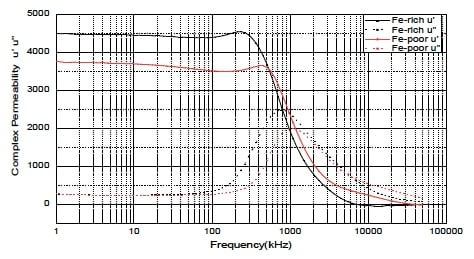Figure 4. u’/u’’~f curve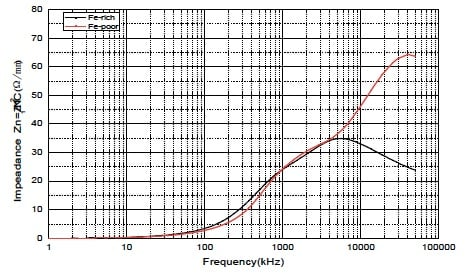Figure 5. Impedance curve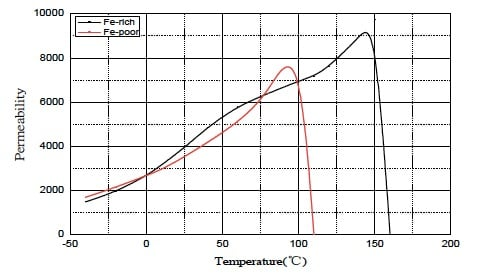Figure 6. Temperature curve

## A Summary of the Fe-poor Composition Formula Experiment Findings

MnZn ferrite with a Fe-poor composition formula can also obtain high permeability, but the Curie temperature is lower than Fe-rich composition formulas. When setting the formula, it is necessary to consider the actual application conditions and choose the ratio of the three main raw materials — Fe2O3, Mn3O4, and ZnO — rationally.

• Share